# Home

 Chiral symmetry of a measured structure 14th November 2015 So far we have developed some important structures based on anharmonic coordinates and spin matrices. If you recall one of our basic assumptions is that all entities that we know or do not know, are in a state of measurement. They are the objects being measured and the observers making measurements simultaneously. The structures we have described in j-space, are invaluable in order to understand the measurements made by observers of varying capacities with respect to each other.  Furthermore these structures are independent of the physical description we are so used to i.e. (t, x, y, z, m, q) reference-frame involving energy and momentum. We know that every macroscopic observer ObsM is situated at Λ∞ plane. The energy and momentum as we use in traditional description, have relevance only to Λ∞ plane.     We have described two very important symmetries (i) Virtual Twin Symmetry (VT-symmetry) (ii) 4π-symmetry. VT-symmetry defines the origin and the quanta. It is validated by the Axiom of Foundation. The 4π-symmetry is the consequence of the application of Pauli Spin Matrices describing a two-state system, to Hamilton's Anharmonic Coordinates which resulted in the unit-point sphere SU. These are the symmetries which are fundamental to the j-space i.e. the measurement spaces for either Joe (q = 3) or Zork (q < 3), both are bound by these symmetries.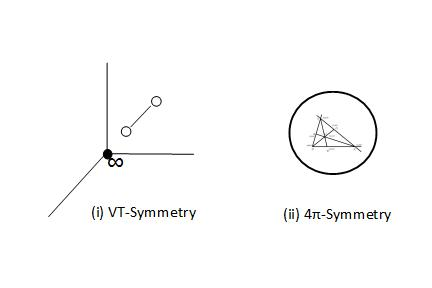However there are also symmetries which define the measured geometrical structures. These secondary symmetries are applicable, only after VT-symmetry and 4π-symmetry are established and the measurement metric has been defined. We declare our ignorance of existence as measured by our senses. Thus we follow in the foot-steps of Kant and develop the description of symmetry in "phenomenon" mode, which incidentally happens to be our universe. We understand that "noumenal" mode is indeterminate and can not be approximated.     In "phenomenon" mode represented by the observer ObsM in Λ∞-plane, the VT-symmetry and 4π-symmetry can not be broken. Further the zero-entropy measurements are not possible in "phenomenon" mode i.e. time-axis must exist on for phenomena. So a symmetry is possible for an observer who can not complete a PE1 measurement in finite-time. Remember time is of consequence only when we try to quantify resources in a observer's measurement frame in terms of momentum and energy. Hence we have VT-symmetry and 4π-symmetry along with time-axis as our guidelines to develop our understanding of what to come, when we (ObsM) start making measurements.     The discrete measurement space or j-space is a space in which no two points are alike which means that no two measurements can be identical unless a symmetry is assumed. This is our own version of Pauli's Exclusion Principle applicable to j-space. Hence theoretically except for VT and 4π symmetries no other symmetry is possible in j-space.     We have discussed earlier that it was the observer's limitations which led to symmetry. Therefore to gain more knowledge the measurements must be made more precise i.e. more resources must be used and that leads to what we call "symmetry-breaking". A symmetry-breaking will always lead to the discovery of new ground-states or entities which are of higher information contents.     Let us envision a measurement apparatus which has capacity for introducing a phase-shift. We will assume that per ObsM the measurement apparatus is perfect i.e. it makes PE1j measurements. To simplify the argument we assume that phase-shift introduced is 180o. We note that apparatus does not have infinite capacity hence it can introduce the phase-shift only in states whose information content is up to a certain limit. The states with higher information content will stay unaffected as the apparatus will not be able to measure them accurately. The phase-shift introduced by the apparatus will be below its measurement threshold for high information states. Clearly if we restricted the capacity of the apparatus to that of the observer ObsM with (t, x, y, z, m, q) metric in Λ∞ plane, it will be analogous to a mirror and the light would be reflected.     We assume that we will be measuring a structure as shown on the left, which consists of unit-point sphere, surface and a macroscopic sphere in SM in (t, x, y, z, m, q) metric. The measurement made by the mirror in this metric, will be an image which consists of SM only. The mirror does not have capacity to measure SU. Actually come to think about it the mirror can not accurately measure Λ∞-plane either. We keep in mind that (t, x, y, z, m, q) metric and Λ∞-plane are not analogous to each other.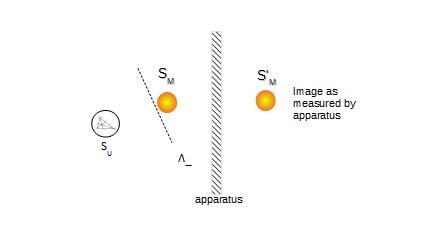In the diagram above, SM and SM' can be superimposed upon each other. The observer will assume a symmetry based on the measurements. Let us now increase the apparatus capacity to measure information i.e. now the new measured entity TM will be heavier than SM. More importantly the relationship between the measured entity TM, Λ∞ and SU will be more explicit and the situation will be more like as shown below: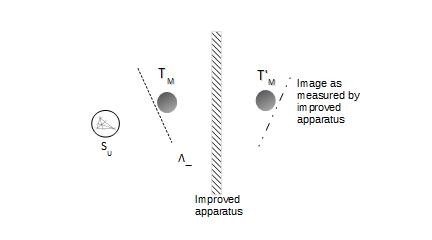Now as we have more information content in the measured structure the situation is very different. Since the image of Λ∞ can not be measured completely, we will be measuring a structure whose image is not identical to it. The unit-sphere SU still can not be measured with improved apparatus. The simple geometrical operations in (t, x, y, z, m, q) metric such as rotation and translations can not transform TM into TM'. The structure measured with diminished capacity is called "achiral" whereas the structure measured with improved capacity is called "chiral". We note that heavier fermions are likely to break chiral symmetry.     The relationship between unit-point sphere SU and the measured structure SM (or TM), is unique and therefore the systems in Λ∞-plane are bound by it. However for low information content structures, complementary systems may be measured by the apparatus. For example as a structure with low information content SM alone can be measured as achiral.     The relationship between unit-point sphere SU  and the measured structure SM is fundamental, independent of the observer's measurement metric. Therefore the structures respecting this relationship, must be measured as the "nature preferred states". These states are likely to follow different interaction mechanism among themselves, than their counterpart image states. An examples is left-handed system vs. right-handed system.     In our description the symmetries appear in order of importance as, (i) VT-symmetry (ii) 4π-symmetry and (iii) chiral. The chiral symmetry can be considered the most elemental symmetry within the observer's measurement metric. Theoretically in pure j-space where no two points are alike, chiral symmetry can not exist. If a state is measured as chiral then there is always another state with higher information content which needs to be discovered by improving the measurement apparatus. Thus the mass generation is an intrinsic property of the j-space as we move towards higher information state by breaking the chiral symmetry. Any other symmetry as observed in (t, x, y, z, m, q) metric in Λ∞-plane will be of lower hierarchy.     Just a note that even if the mirror symmetry does not hold then a group of entities can still be measured as identical, which is true for any particle with low information content. The symmetry which allows it, is VT-symmetry. Examples are electrons and photons in q = 3 space in Λ∞-plane.     Finally we do not know of a particle which is completely unique. Obsi-criterion demands it but ObsM can not measure it in j-space. Can we think of an example of such entity based on the diagrams in this blog? Think "root"!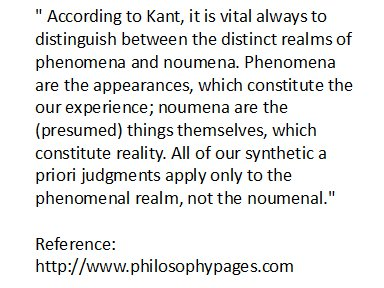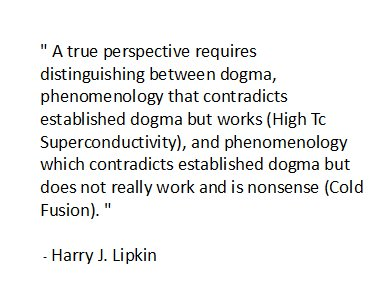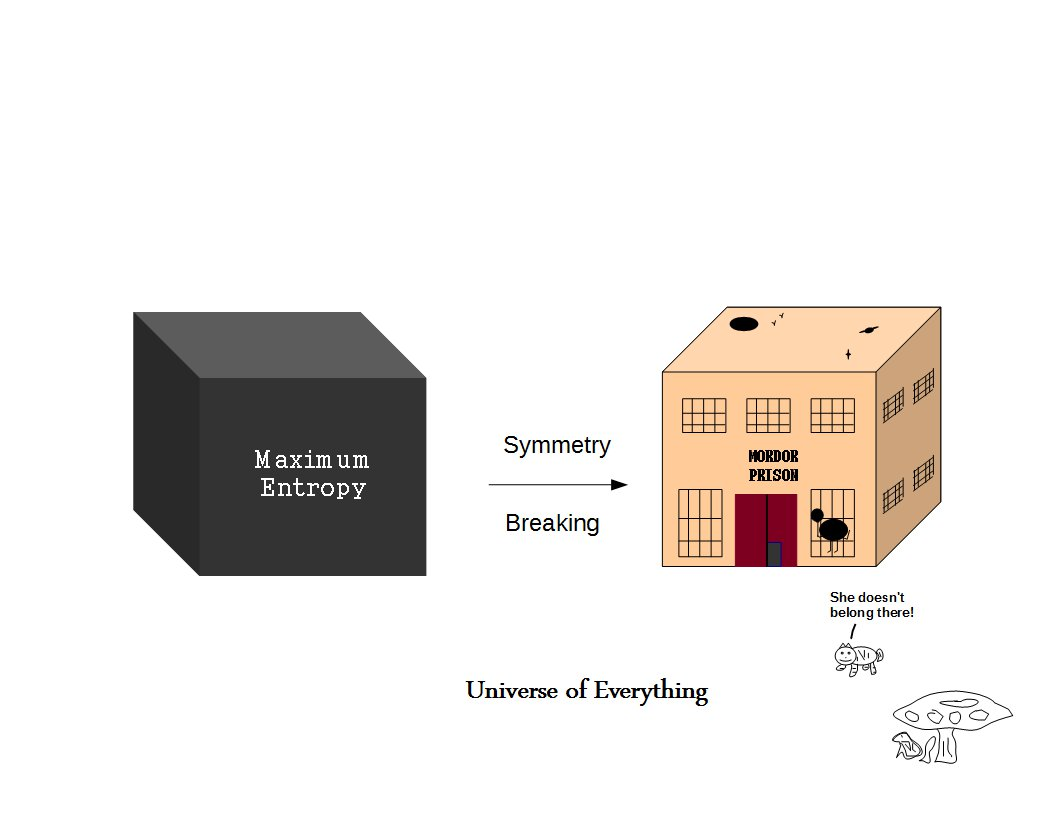Best viewed with Internet Explorer